Скачать презентацию FMRI Analysis Experiment Design Scanning Pre-Processing Individual Subject

67a5f913b081d579c78ac04601a6d874.ppt

• Количество слайдов: 29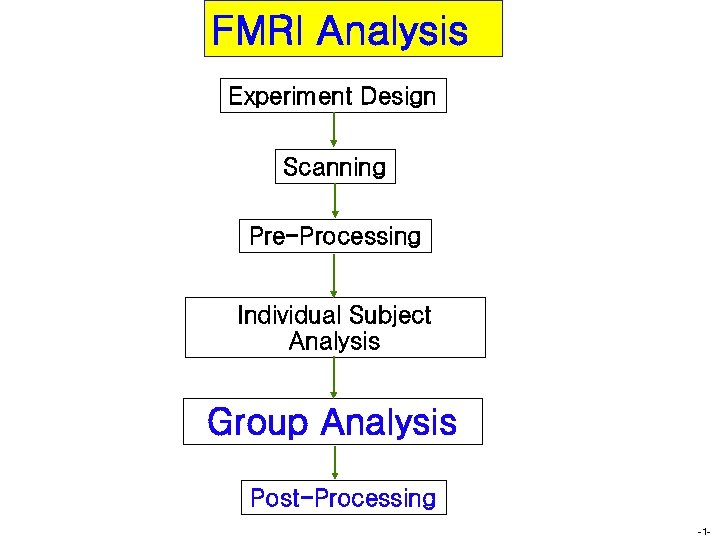FMRI Analysis Experiment Design Scanning Pre-Processing Individual Subject Analysis Group Analysis Post-Processing -1 -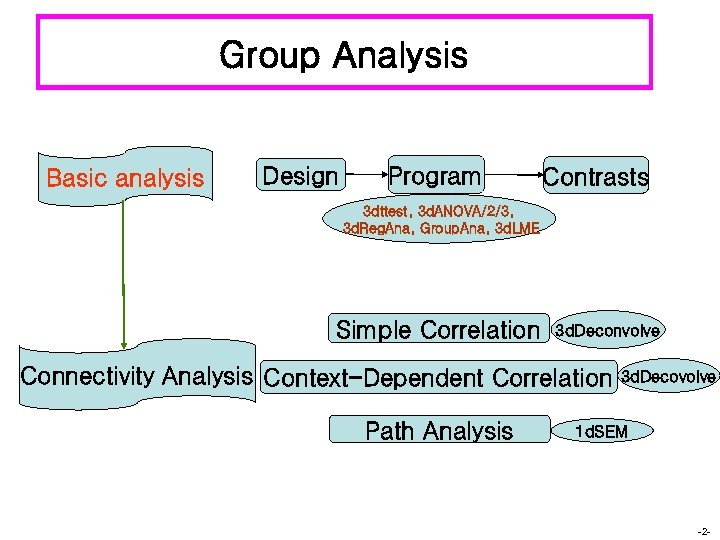Group Analysis Basic analysis Design Program Contrasts 3 dttest, 3 d. ANOVA/2/3, 3 d. Reg. Ana, Group. Ana, 3 d. LME Simple Correlation 3 d. Deconvolve Connectivity Analysis Context-Dependent Correlation Path Analysis 3 d. Decovolve 1 d. SEM -2 -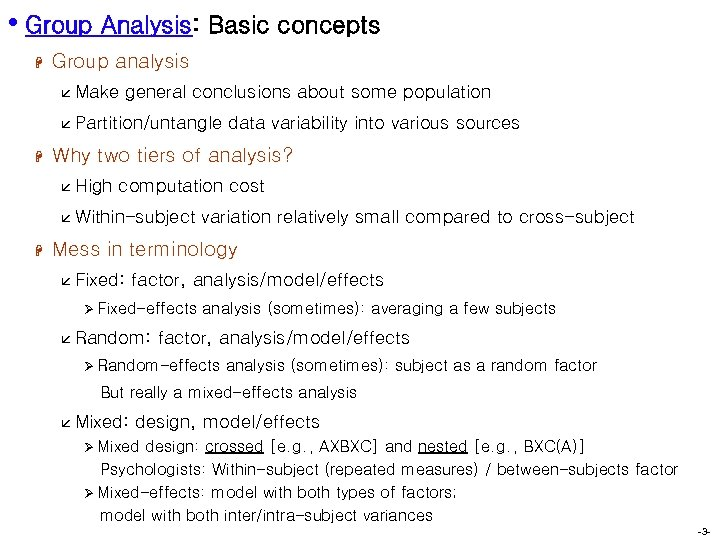• Group Analysis: Basic concepts H Group analysis å Make general conclusions about some population å Partition/untangle H Why two tiers of analysis? å High computation cost å Within-subject H data variability into various sources variation relatively small compared to cross-subject Mess in terminology å Fixed: factor, analysis/model/effects Ø Fixed-effects å Random: analysis (sometimes): averaging a few subjects factor, analysis/model/effects Ø Random-effects analysis (sometimes): subject as a random factor But really a mixed-effects analysis å Mixed: design, model/effects Ø Mixed design: crossed [e. g. , AXBXC] and nested [e. g. , BXC(A)] Psychologists: Within-subject (repeated measures) / between-subjects factor Ø Mixed-effects: model with both types of factors; model with both inter/intra-subject variances -3 -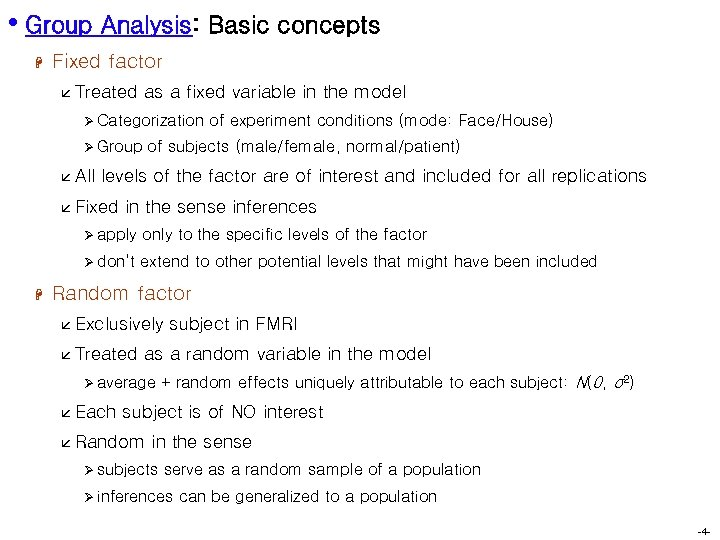• Group Analysis: Basic concepts H Fixed factor å Treated as a fixed variable in the model Ø Categorization Ø Group å All of experiment conditions (mode: Face/House) of subjects (male/female, normal/patient) levels of the factor are of interest and included for all replications å Fixed in the sense inferences Ø apply Ø don’t H only to the specific levels of the factor extend to other potential levels that might have been included Random factor å Exclusively å Treated as a random variable in the model Ø average å Each subject in FMRI + random effects uniquely attributable to each subject: N(0, σ2) subject is of NO interest å Random in the sense Ø subjects serve as a random sample of a population Ø inferences can be generalized to a population -4 -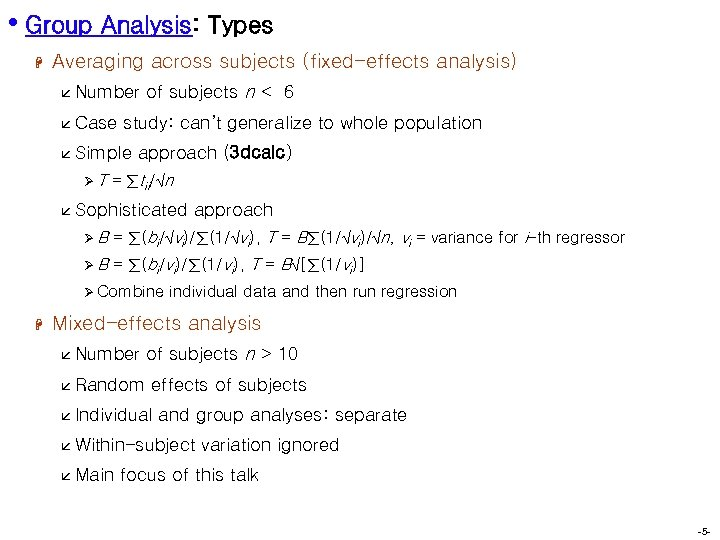• Group Analysis: Types H Averaging across subjects (fixed-effects analysis) å Number å Case study: can’t generalize to whole population å Simple ØT of subjects n < 6 approach (3 dcalc) = ∑tii/√n å Sophisticated approach ØB = ∑(bi/√vi)/∑(1/√vi), T = B∑(1/√vi)/√n, vi = variance for i-th regressor ØB = ∑(bi/vi)/∑(1/vi), T = B√[∑(1/vi)] Ø Combine H individual data and then run regression Mixed-effects analysis å Number å Random of subjects n > 10 effects of subjects å Individual and group analyses: separate å Within-subject å Main variation ignored focus of this talk -5 -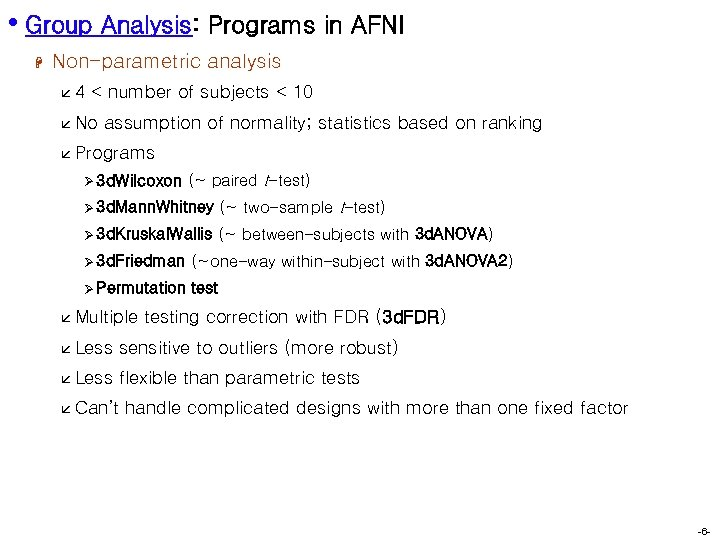• Group Analysis: Programs in AFNI H Non-parametric analysis å 4 < number of subjects < 10 å No assumption of normality; statistics based on ranking å Programs Ø 3 d. Wilcoxon (~ paired t-test) Ø 3 d. Mann. Whitney (~ two-sample t-test) Ø 3 d. Kruskal. Wallis (~ between-subjects with 3 d. ANOVA) Ø 3 d. Friedman (~one-way within-subject with 3 d. ANOVA 2) Ø Permutation test å Multiple testing correction with FDR (3 d. FDR) å Less sensitive to outliers (more robust) å Less flexible than parametric tests å Can’t handle complicated designs with more than one fixed factor -6 -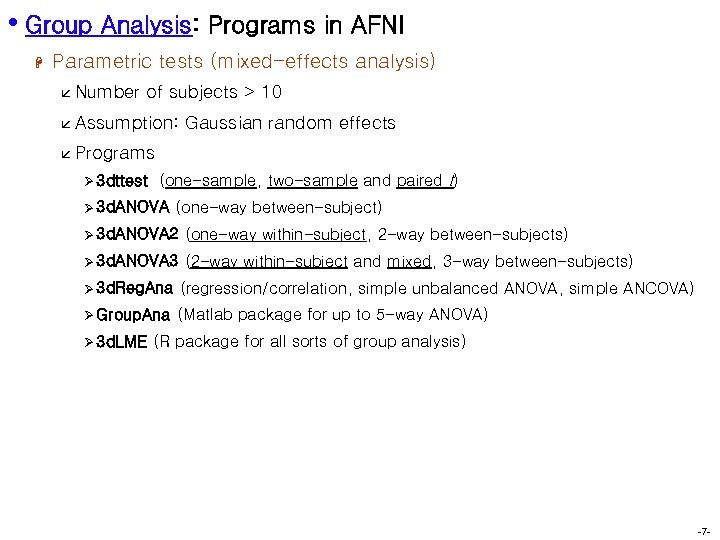• Group Analysis: Programs in AFNI H Parametric tests (mixed-effects analysis) å Number of subjects > 10 å Assumption: Gaussian random effects å Programs Ø 3 dttest (one-sample, two-sample and paired t) Ø 3 d. ANOVA (one-way between-subject) Ø 3 d. ANOVA 2 (one-way within-subject, 2 -way between-subjects) Ø 3 d. ANOVA 3 (2 -way within-subject and mixed, 3 -way between-subjects) Ø 3 d. Reg. Ana (regression/correlation, simple unbalanced ANOVA, simple ANCOVA) Ø Group. Ana (Matlab package for up to 5 -way ANOVA) Ø 3 d. LME (R package for all sorts of group analysis) -7 -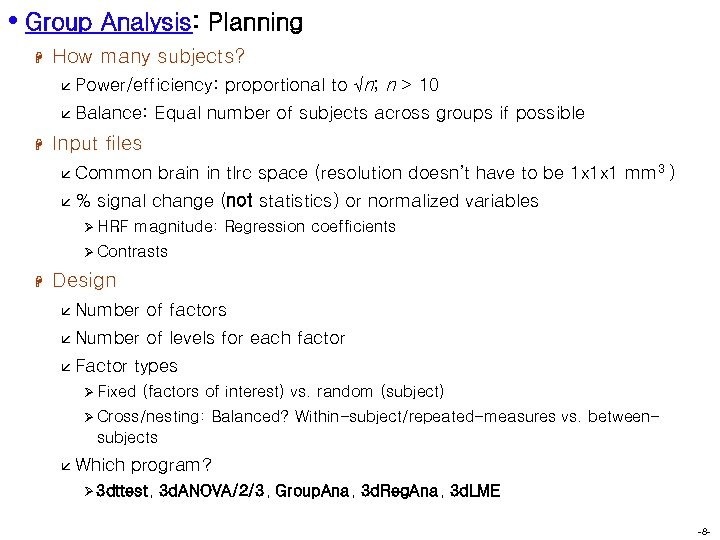• Group Analysis: Planning H How many subjects? å Power/efficiency: å Balance: H proportional to √n; n > 10 Equal number of subjects across groups if possible Input files å Common å% brain in tlrc space (resolution doesn’t have to be 1 x 1 x 1 mm 3 ) signal change (not statistics) or normalized variables Ø HRF magnitude: Regression coefficients Ø Contrasts H Design å Number of factors å Number of levels for each factor å Factor types Ø Fixed (factors of interest) vs. random (subject) Ø Cross/nesting: Balanced? Within-subject/repeated-measures vs. between- subjects å Which program? Ø 3 dttest, 3 d. ANOVA/2/3, Group. Ana, 3 d. Reg. Ana, 3 d. LME -8 -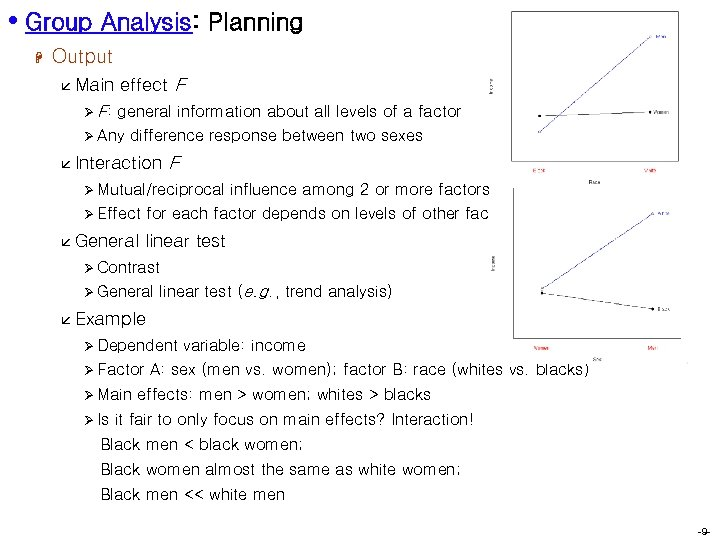• Group Analysis: Planning H Output å Main Ø F: effect F general information about all levels of a factor Ø Any difference response between two sexes å Interaction F Ø Mutual/reciprocal Ø Effect å General influence among 2 or more factors for each factor depends on levels of other factors linear test Ø Contrast Ø General linear test (e. g. , trend analysis) å Example Ø Dependent Ø Factor Ø Main Ø Is variable: income A: sex (men vs. women); factor B: race (whites vs. blacks) effects: men > women; whites > blacks it fair to only focus on main effects? Interaction! Black men < black women; Black women almost the same as white women; Black men << white men -9 -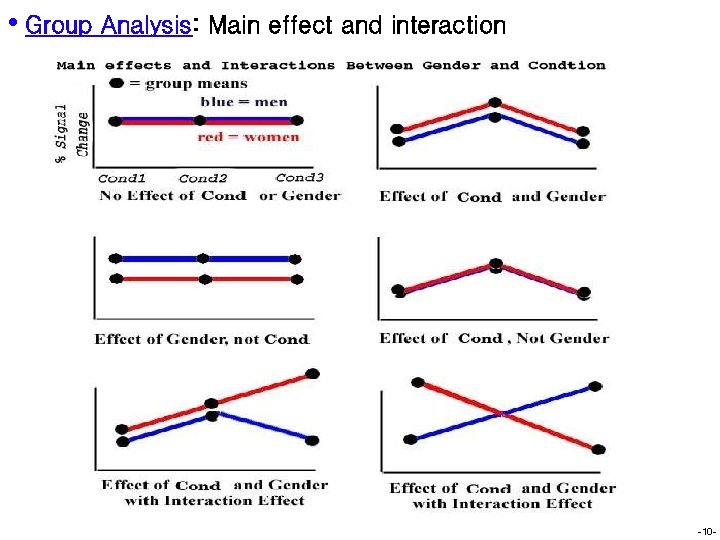• Group Analysis: Main effect and interaction -10 -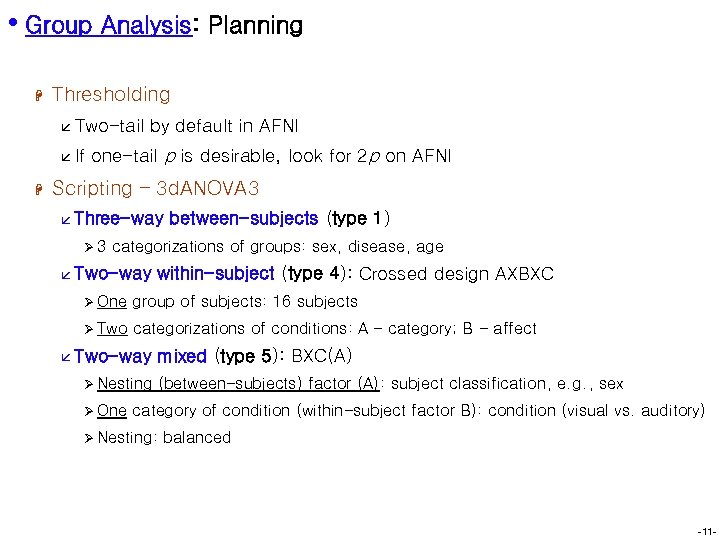• Group Analysis: Planning H Thresholding å Two-tail å If H by default in AFNI one-tail p is desirable, look for 2 p on AFNI Scripting – 3 d. ANOVA 3 å Three-way Ø 3 between-subjects (type 1) categorizations of groups: sex, disease, age å Two-way within-subject (type 4): Crossed design AXBXC Ø One group of subjects: 16 subjects Ø Two categorizations of conditions: A – category; B - affect å Two-way Ø Nesting Ø One mixed (type 5): BXC(A) (between-subjects) factor (A): subject classification, e. g. , sex category of condition (within-subject factor B): condition (visual vs. auditory) Ø Nesting: balanced -11 -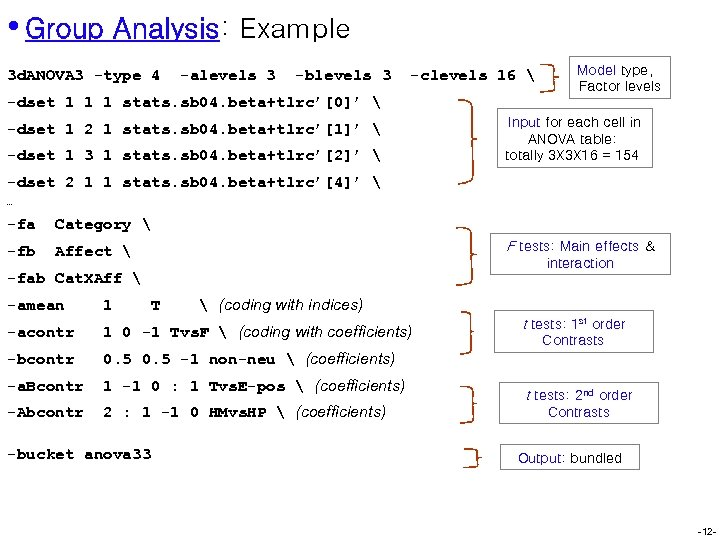• Group Analysis: Example 3 d. ANOVA 3 -type 4 -alevels 3 -blevels 3 -clevels 16 Model type, Factor levels -dset 1 1 1 stats. sb 04. beta+tlrc’’ -dset 1 2 1 stats. sb 04. beta+tlrc’’ -dset 1 3 1 stats. sb 04. beta+tlrc’’ Input for each cell in ANOVA table: totally 3 X 3 X 16 = 154 -dset 2 1 1 stats. sb 04. beta+tlrc’’ … -fa Category -fb Affect F tests: Main effects & interaction -fab Cat. XAff 1 -acontr 1 0 -1 Tvs. F (coding with coefficients) -bcontr 0. 5 -1 non-neu (coefficients) -a. Bcontr 1 -1 0 : 1 Tvs. E-pos (coefficients) -Abcontr 2 : 1 -1 0 HMvs. HP (coefficients) -bucket anova 33 T (coding with indices) -amean t tests: 1 st order Contrasts t tests: 2 nd order Contrasts Output: bundled -12 -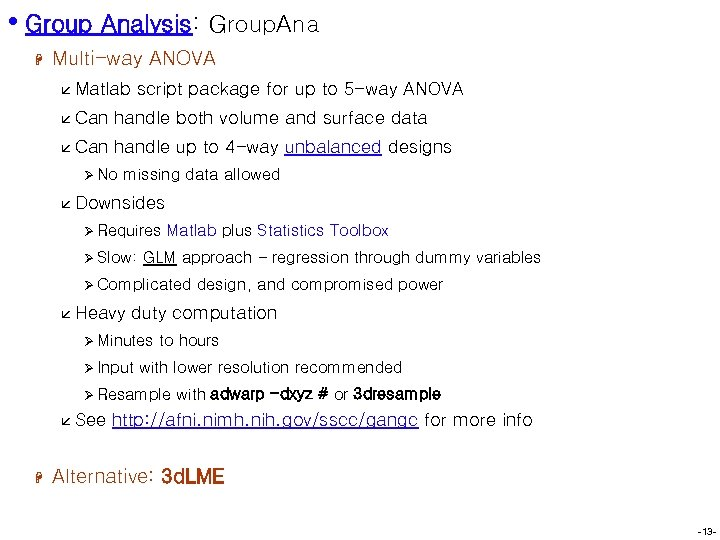• Group Analysis: Group. Ana H Multi-way ANOVA å Matlab script package for up to 5 -way ANOVA å Can handle both volume and surface data å Can handle up to 4 -way unbalanced designs Ø No missing data allowed å Downsides Ø Requires Ø Slow: Matlab plus Statistics Toolbox GLM approach - regression through dummy variables Ø Complicated å Heavy duty computation Ø Minutes Ø Input to hours with lower resolution recommended Ø Resample å See H design, and compromised power with adwarp -dxyz # or 3 dresample http: //afni. nimh. nih. gov/sscc/gangc for more info Alternative: 3 d. LME -13 -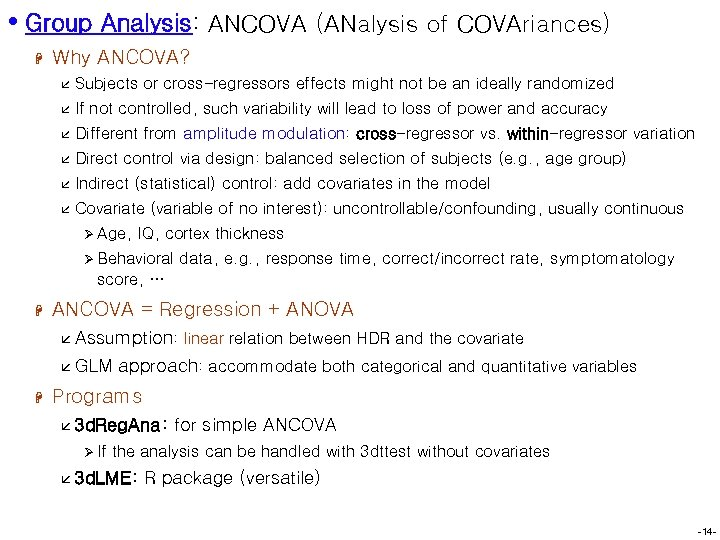• Group Analysis: ANCOVA (ANalysis of COVAriances) H Why ANCOVA? å Subjects or cross-regressors effects might not be an ideally randomized å If not controlled, such variability will lead to loss of power and accuracy å Different from amplitude modulation: cross-regressor vs. within-regressor variation å Direct control via design: balanced selection of subjects (e. g. , age group) å Indirect (statistical) control: add covariates in the model å Covariate (variable of no interest): uncontrollable/confounding, usually continuous Ø Age, IQ, cortex thickness Ø Behavioral data, e. g. , response time, correct/incorrect rate, symptomatology score, … H ANCOVA = Regression + ANOVA å Assumption: å GLM H linear relation between HDR and the covariate approach: accommodate both categorical and quantitative variables Programs å 3 d. Reg. Ana: Ø If for simple ANCOVA the analysis can be handled with 3 dttest without covariates å 3 d. LME: R package (versatile) -14 -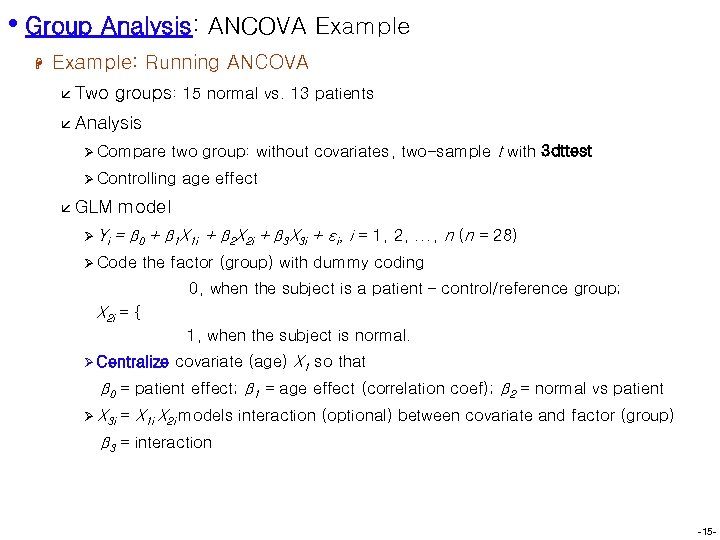• Group Analysis: ANCOVA Example H Example: Running ANCOVA å Two groups: 15 normal vs. 13 patients å Analysis Ø Compare two group: without covariates, two-sample t with 3 dttest Ø Controlling å GLM Ø Yi age effect model = β 0 + β 1 X 1 i + β 2 X 2 i + β 3 X 3 i + εi, i = 1, 2, . . . , n (n = 28) Ø Code the factor (group) with dummy coding 0, when the subject is a patient – control/reference group; X 2 i = { 1, when the subject is normal. Ø Centralize covariate (age) X 1 so that β 0 = patient effect; β 1 = age effect (correlation coef); β 2 = normal vs patient Ø X 3 i = X 1 i X 2 i models interaction (optional) between covariate and factor (group) β 3 = interaction -15 -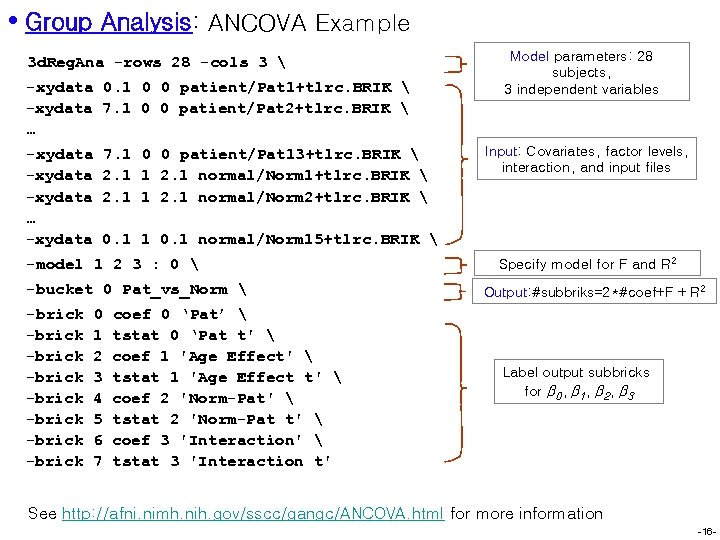• Group Analysis: ANCOVA Example 3 d. Reg. Ana -rows 28 -cols 3 -xydata 0. 1 0 0 patient/Pat 1+tlrc. BRIK -xydata 7. 1 0 0 patient/Pat 2+tlrc. BRIK … -xydata … -xydata 7. 1 0 0 patient/Pat 13+tlrc. BRIK 2. 1 1 2. 1 normal/Norm 1+tlrc. BRIK 2. 1 1 2. 1 normal/Norm 2+tlrc. BRIK Input: Covariates, factor levels, interaction, and input files 0. 1 1 0. 1 normal/Norm 15+tlrc. BRIK -model 1 2 3 : 0 -bucket 0 Pat_vs_Norm -brick -brick Model parameters: 28 subjects, 3 independent variables 0 1 2 3 4 5 6 7 coef 0 ‘Pat’ tstat 0 ‘Pat t' coef 1 'Age Effect' tstat 1 'Age Effect t' coef 2 'Norm-Pat' tstat 2 'Norm-Pat t' coef 3 'Interaction' tstat 3 'Interaction t' Specify model for F and R 2 Output: #subbriks=2*#coef+F + R 2 Label output subbricks for β 0, β 1, β 2, β 3 See http: //afni. nimh. nih. gov/sscc/gangc/ANCOVA. html for more information -16 -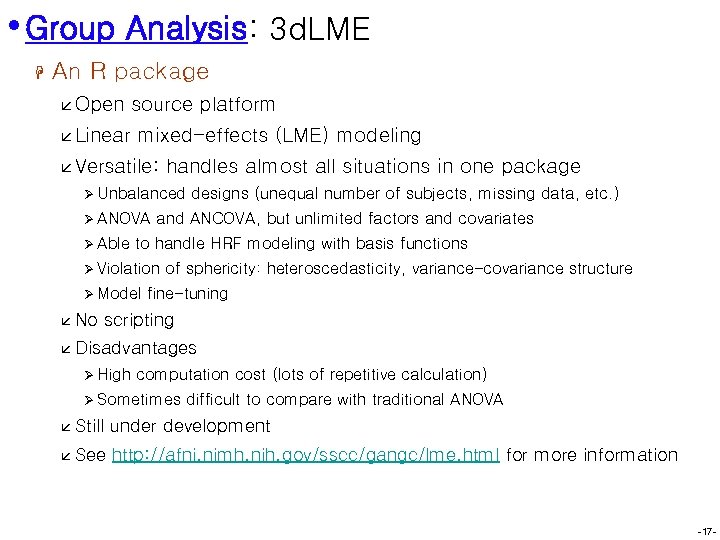• Group Analysis: 3 d. LME H An R package å Open å Linear source platform mixed-effects (LME) modeling å Versatile: handles almost all situations in one package Ø Unbalanced Ø ANOVA Ø Able and ANCOVA, but unlimited factors and covariates to handle HRF modeling with basis functions Ø Violation Ø Model å No designs (unequal number of subjects, missing data, etc. ) of sphericity: heteroscedasticity, variance-covariance structure fine-tuning scripting å Disadvantages Ø High computation cost (lots of repetitive calculation) Ø Sometimes difficult to compare with traditional ANOVA å Still under development å See http: //afni. nimh. nih. gov/sscc/gangc/lme. html for more information -17 -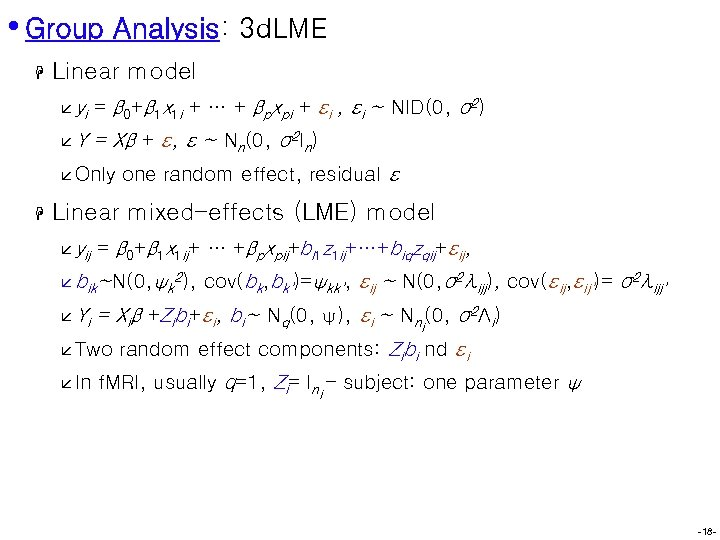• Group Analysis: 3 d. LME H Linear model å yi = β 0+β 1 x 1 i + … + βpxpi + εi , εi ~ NID(0, σ2) åY = Xβ + ε, ε ~ Nn(0, σ2 In) å Only H one random effect, residual ε Linear mixed-effects (LME) model å yij = β 0+β 1 x 1 ij+ … +βpxpij+bi 1 z 1 ij+…+biqzqij+εij, å bik~N(0, ψk 2), å Yi = Xiβ +Zibi+εi, bi~ Nq(0, ψ), εi ~ Nni(0, σ2Λi) å Two å In cov(bk, bk’)=ψkk’, εij ~ N(0, σ2λijj), cov(εij, εij’)= σ2λijj’ random effect components: Zibi nd εi f. MRI, usually q=1, Zi= Ini – subject: one parameter ψ -18 -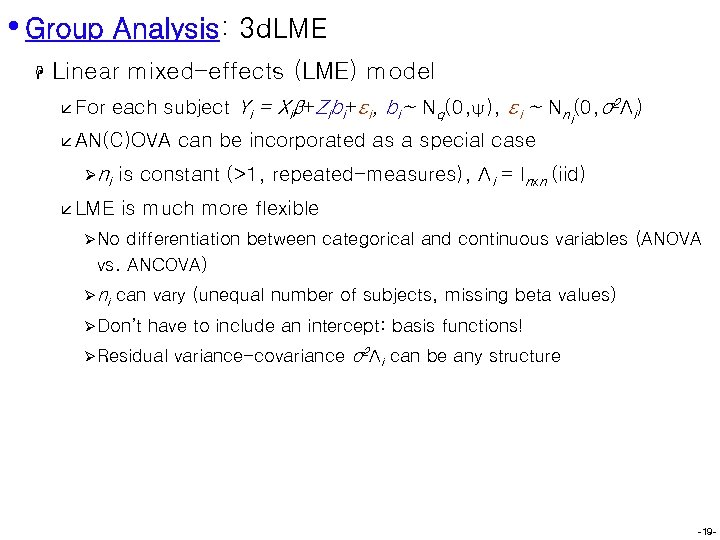• Group Analysis: 3 d. LME H Linear mixed-effects (LME) model å For each subject Yi = Xiβ+Zibi+εi, bi~ Nq(0, ψ), εi ~ Nni(0, σ2Λi) å AN(C)OVA Ø ni can be incorporated as a special case is constant (>1, repeated-measures), Λi = Inxn (iid) å LME is much more flexible Ø No differentiation between categorical and continuous variables (ANOVA vs. ANCOVA) Ø ni can vary (unequal number of subjects, missing beta values) Ø Don’t have to include an intercept: basis functions! Ø Residual variance-covariance σ2Λi can be any structure -19 -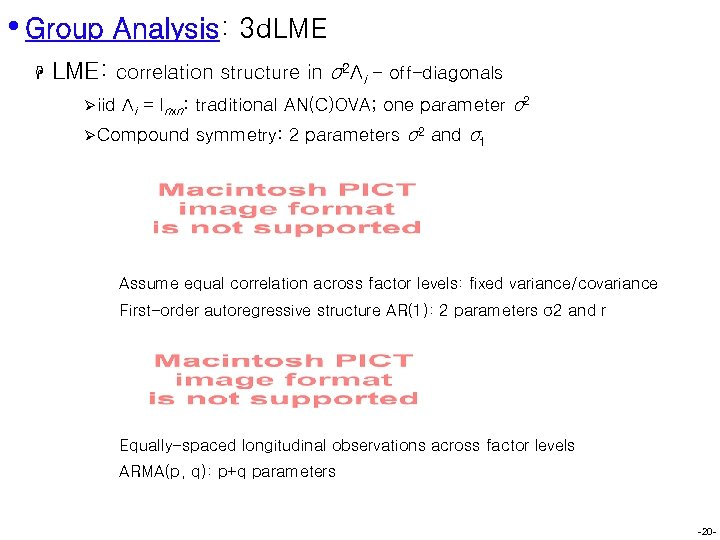• Group Analysis: 3 d. LME H LME: correlation structure in σ2Λi - off-diagonals Ø iid Λi = Inxn: traditional AN(C)OVA; one parameter σ2 Ø Compound symmetry: 2 parameters σ2 and σ1 Assume equal correlation across factor levels: fixed variance/covariance First-order autoregressive structure AR(1): 2 parameters σ2 and r Equally-spaced longitudinal observations across factor levels ARMA(p, q): p+q parameters -20 -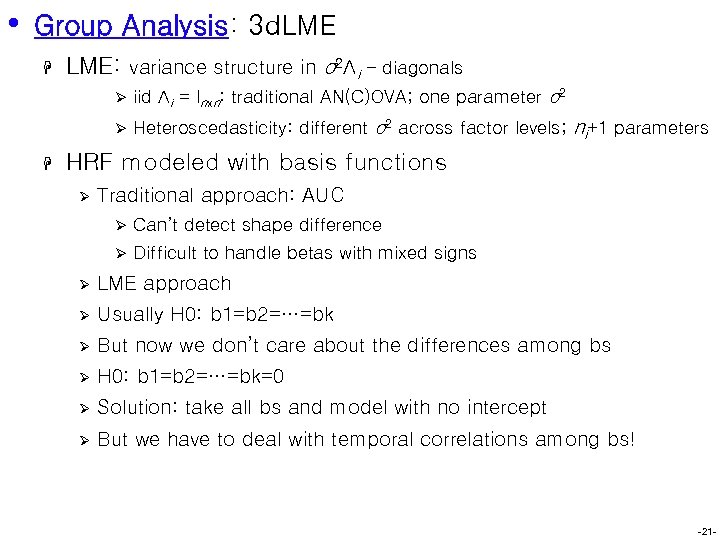• Group Analysis: 3 d. LME H LME: variance structure in σ2Λi - diagonals Ø Ø H iid Λi = Inxn: traditional AN(C)OVA; one parameter σ2 Heteroscedasticity: different σ2 across factor levels; ni+1 parameters HRF modeled with basis functions Ø Traditional approach: AUC Ø Can’t detect shape difference Ø Difficult to handle betas with mixed signs Ø LME approach Ø Usually H 0: b 1=b 2=…=bk Ø But now we don’t care about the differences among bs Ø H 0: b 1=b 2=…=bk=0 Ø Solution: take all bs and model with no intercept Ø But we have to deal with temporal correlations among bs! -21 -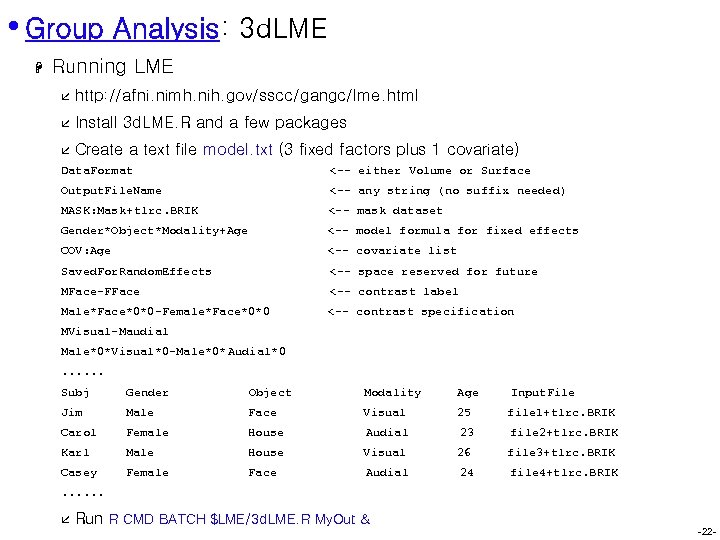• Group Analysis: 3 d. LME H Running LME å http: //afni. nimh. nih. gov/sscc/gangc/lme. html å Install 3 d. LME. R and a few packages å Create a text file model. txt (3 fixed factors plus 1 covariate) Data. Format <-- either Volume or Surface Output. File. Name <-- any string (no suffix needed) MASK: Mask+tlrc. BRIK <-- mask dataset Gender*Object*Modality+Age <-- model formula for fixed effects COV: Age <-- covariate list Saved. For. Random. Effects <-- space reserved for future MFace-FFace <-- contrast label Male*Face*0*0 -Female*Face*0*0 <-- contrast specification MVisual-Maudial Male*0*Visual*0 -Male*0*Audial*0. . . Subj Gender Object Modality Age Input. File Jim Male Face Visual 25 file 1+tlrc. BRIK Carol Female House Audial 23 file 2+tlrc. BRIK Karl Male House Visual 26 file 3+tlrc. BRIK Casey Female Face Audial 24 file 4+tlrc. BRIK . . . å Run R CMD BATCH \$LME/3 d. LME. R My. Out & -22 -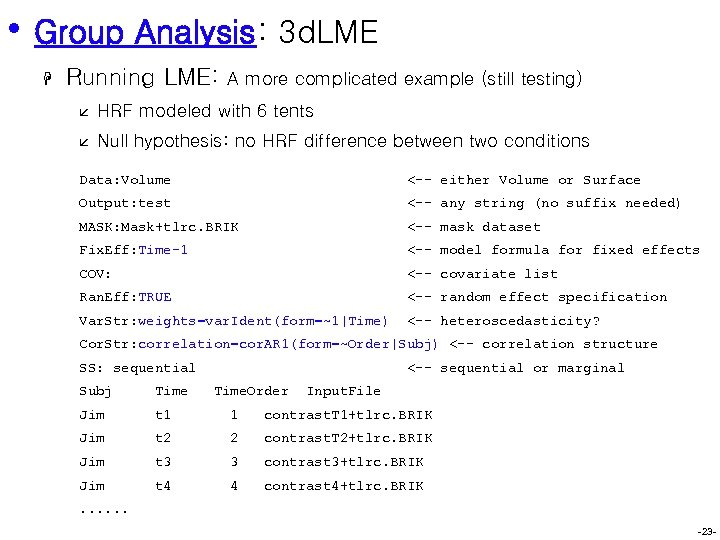• Group Analysis: 3 d. LME H Running LME: A more complicated example (still testing) å HRF modeled with 6 tents å Null hypothesis: no HRF difference between two conditions Data: Volume <-- either Volume or Surface Output: test <-- any string (no suffix needed) MASK: Mask+tlrc. BRIK <-- mask dataset Fix. Eff: Time-1 <-- model formula for fixed effects COV: <-- covariate list Ran. Eff: TRUE <-- random effect specification Var. Str: weights=var. Ident(form=~1|Time) <-- heteroscedasticity? Cor. Str: correlation=cor. AR 1(form=~Order|Subj) <-- correlation structure SS: sequential <-- sequential or marginal Subj Time. Order Input. File Jim t 1 1 contrast. T 1+tlrc. BRIK Jim t 2 2 contrast. T 2+tlrc. BRIK Jim t 3 3 contrast 3+tlrc. BRIK Jim t 4 4 contrast 4+tlrc. BRIK . . . -23 -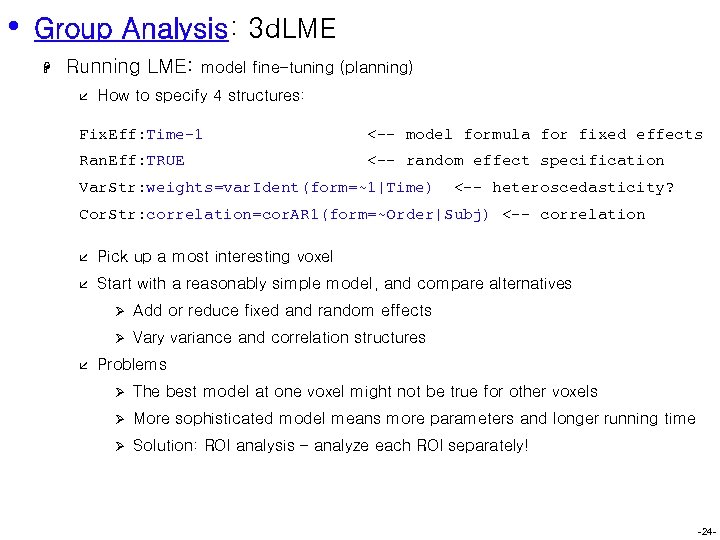• Group Analysis: 3 d. LME H Running LME: å model fine-tuning (planning) How to specify 4 structures: Fix. Eff: Time-1 <-- model formula for fixed effects Ran. Eff: TRUE <-- random effect specification Var. Str: weights=var. Ident(form=~1|Time) <-- heteroscedasticity? Cor. Str: correlation=cor. AR 1(form=~Order|Subj) <-- correlation å Pick up a most interesting voxel å Start with a reasonably simple model, and compare alternatives Ø Ø å Add or reduce fixed and random effects Vary variance and correlation structures Problems Ø The best model at one voxel might not be true for other voxels Ø More sophisticated model means more parameters and longer running time Ø Solution: ROI analysis – analyze each ROI separately! -24 -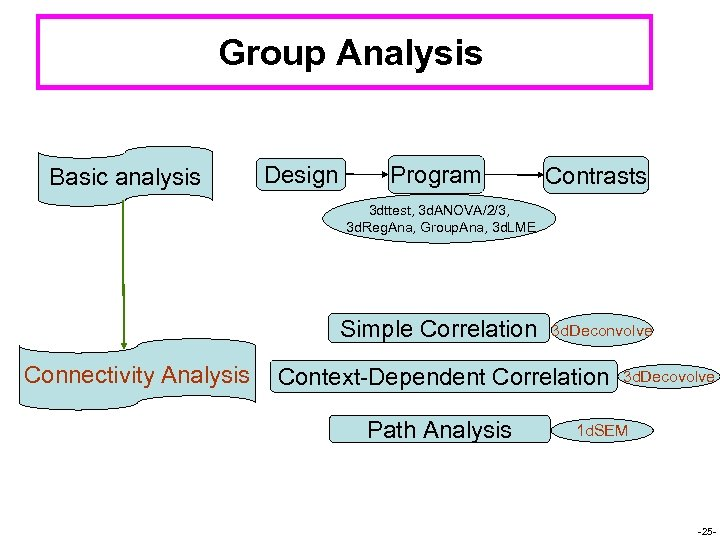Group Analysis Basic analysis Design Program Contrasts 3 dttest, 3 d. ANOVA/2/3, 3 d. Reg. Ana, Group. Ana, 3 d. LME Simple Correlation Connectivity Analysis 3 d. Deconvolve Context-Dependent Correlation Path Analysis 3 d. Decovolve 1 d. SEM -25 -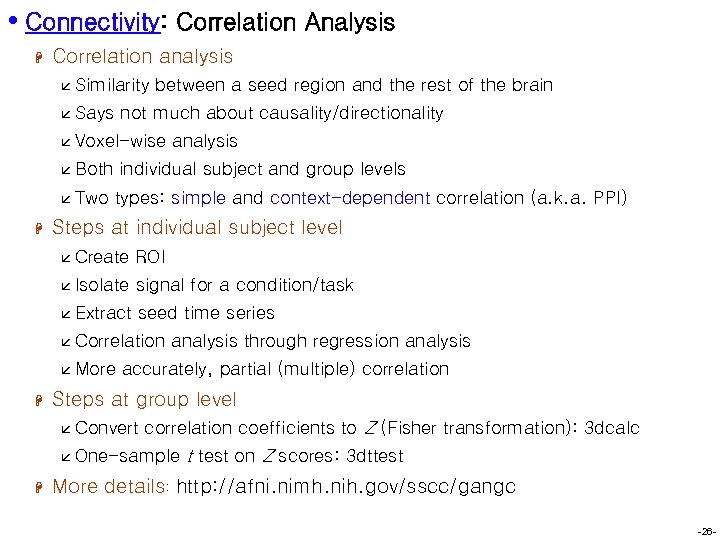• Connectivity: Correlation Analysis H Correlation analysis å Similarity å Says between a seed region and the rest of the brain not much about causality/directionality å Voxel-wise å Both å Two H analysis individual subject and group levels types: simple and context-dependent correlation (a. k. a. PPI) Steps at individual subject level å Create ROI å Isolate signal for a condition/task å Extract seed time series å Correlation å More H analysis through regression analysis accurately, partial (multiple) correlation Steps at group level å Convert correlation coefficients to Z (Fisher transformation): 3 dcalc å One-sample H t test on Z scores: 3 dttest More details: http: //afni. nimh. nih. gov/sscc/gangc -26 -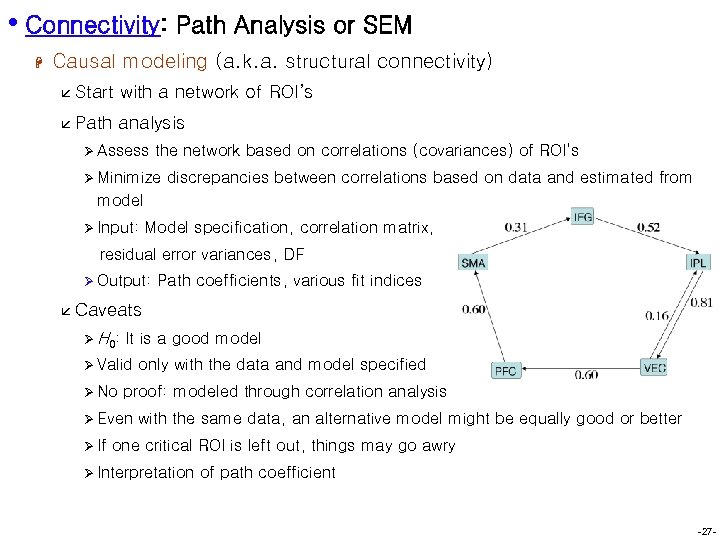• Connectivity: Path Analysis or SEM H Causal modeling (a. k. a. structural connectivity) å Start with a network of ROI’s å Path analysis Ø Assess the network based on correlations (covariances) of ROI’s Ø Minimize discrepancies between correlations based on data and estimated from model Ø Input: Model specification, correlation matrix, residual error variances, DF Ø Output: Path coefficients, various fit indices å Caveats Ø H 0: It is a good model Ø Valid Ø No proof: modeled through correlation analysis Ø Even Ø If only with the data and model specified with the same data, an alternative model might be equally good or better one critical ROI is left out, things may go awry Ø Interpretation of path coefficient -27 -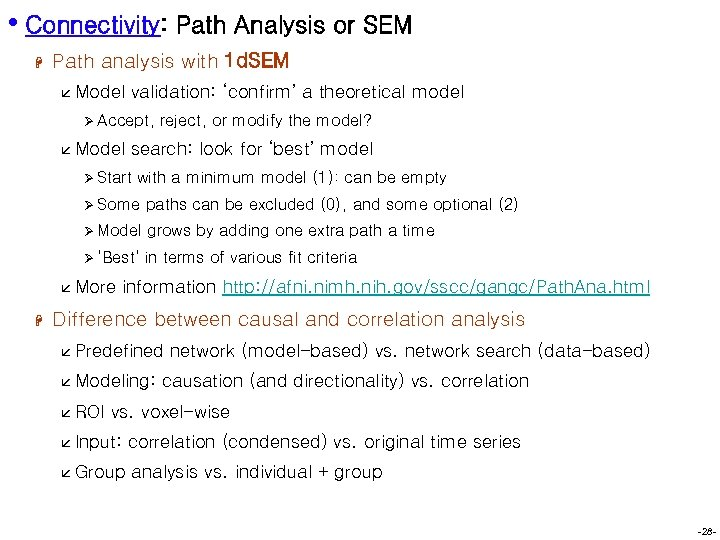• Connectivity: Path Analysis or SEM H Path analysis with 1 d. SEM å Model validation: ‘confirm’ a theoretical model Ø Accept, å Model reject, or modify the model? search: look for ‘best’ model Ø Start with a minimum model (1): can be empty Ø Some paths can be excluded (0), and some optional (2) Ø Model grows by adding one extra path a time Ø ‘Best’ in terms of various fit criteria å More H information http: //afni. nimh. nih. gov/sscc/gangc/Path. Ana. html Difference between causal and correlation analysis å Predefined å Modeling: å ROI network (model-based) vs. network search (data-based) causation (and directionality) vs. correlation vs. voxel-wise å Input: correlation (condensed) vs. original time series å Group analysis vs. individual + group -28 -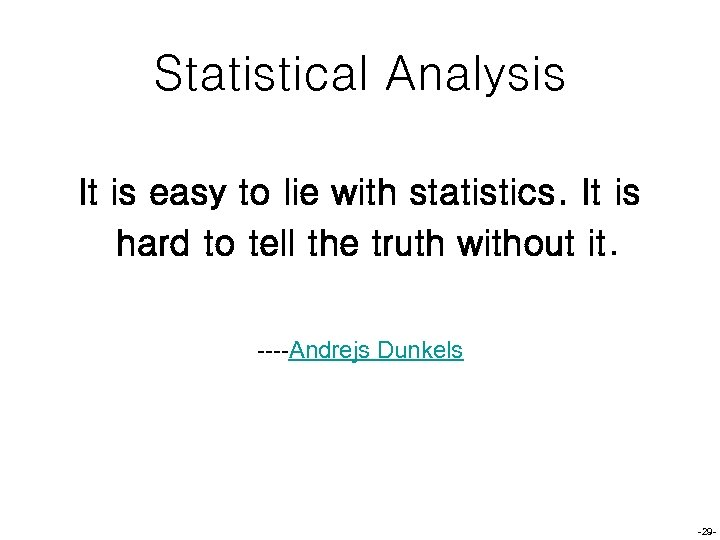Statistical Analysis It is easy to lie with statistics. It is hard to tell the truth without it. ----Andrejs Dunkels -29 -